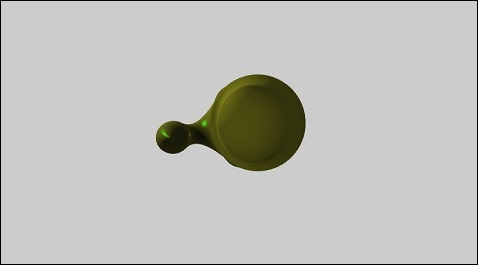# BabylonJS - Tube

Tube is a curved cylinder shape. It can give different parametric shapes based on the equation (maths function) applied to it to get the co-ordinates.

## Syntax

Following is the syntax to create a tube −

```var tube =  BABYLON.Mesh.CreateTube(name, path, radius, tessellation, radiusFunction, cap, scene, updatable?, sideOrientation);
```

## Parameters

Consider the following parameters to create a tube −

• Name − The name given to the tube.

• Path − It is the path on which the tube will be constructed. This path is the central axis of the tube. This array must have at least two Vector3. The first point is the start of the tube and the last point is the end of the tube. So having only two points, you get a simple cylinder.

• Radius − This is the constant radius value applied along the tube. This value is taken into account only if the parameter is radiusFunction null.

• Tessellation − This is related to the number of radial segments. If you set it to 3, you get a triangular tube section; if you set it to 4, you get a squared section, and so on. So set it to the level of precision you need; the more segments, the heavier your mesh.

• RadiusFunction − A custom javascript function. This function will be called at each point of the path while constructing the tube. It will take 2 arguments, the position of the current point and the distance of this point from the start. The function will return the radius based on calculation.

• Cap − BABYLON.Mesh.NO_CAP, BABYLON.Mesh.CAP_START, BABYLON.Mesh.CAP_END, BABYLON.Mesh.CAP_ALL.

• Scene − The scene where the tube will be shown.

• Updatable − By default, this is set to false. If set to true, the mesh can be updatable.

• SideOrientation − It takes the default side orientation.

```var myradiusFunction = function(i, distance) {
var radius = 3 * Math.cos(distance / 5);
};
var tube = BABYLON.Mesh.CreateTube("tubr", path, null, 20, myFunction, scene);
```

## Demo

```<!doctype html>
<html>
<meta charset = "utf-8">
<title>BabylonJs - Basic Element-Creating Scene</title>
<script src = "babylon.js"></script>
<style>
canvas {width: 100%; height: 100%;}
</style>

<body>
<canvas id = "renderCanvas"></canvas>
<script type = "text/javascript">
var canvas = document.getElementById("renderCanvas");
var engine = new BABYLON.Engine(canvas, true);
var createScene  = function() {

var scene = new BABYLON.Scene(engine);
scene.clearColor = new BABYLON.Color3(0.8, 0.8, 0.8);
var camera = new BABYLON.ArcRotateCamera("Camera", 3 *Math.PI / 2, Math.PI / 2, 50, BABYLON.Vector3.Zero(), scene);
camera.attachControl(canvas, false);

// lights
var light = new BABYLON.HemisphericLight("hemi", new BABYLON.Vector3(0, 1, 0), scene);
light.groundColor = new BABYLON.Color3(0.2, 0.2, 0.5);
light.intensity = 0.6;

var light2 = new BABYLON.PointLight("light2", new BABYLON.Vector3(-20, 0, -20), scene);
light2.diffuse = BABYLON.Color3.White();
light2.specular = BABYLON.Color3.Green();
light2.intensity = 0.6;

var mat = new BABYLON.StandardMaterial("mat1", scene);
mat.alpha = 1.0;
mat.diffuseColor = new BABYLON.Color3(0.5, 0.5, 0.1);
mat.backFaceCulling = false;
mat.wireframe = false;

var curvePoints = function(l, t) {
// mathematical function to calculate the curve points.
var path = [];
var step = l / t;
var a = 5;
for (var i = -l/2; i < l/2; i += step ) {
path.push( new BABYLON.Vector3(5 * Math.sin(i*t / 400), 5 * Math.cos(i*t / 400), 0) );
}
return path;
};

var curve = curvePoints(10, 150);

var radiusFunction = function(i, distance) {
// function to calculate the radiusfunction.
var t = i / Math.PI * 2 / 8;
var radius =  Math.sin(t) + i / 25;
};

var tube = BABYLON.Mesh.CreateTube("tube", curve, 2, 60, radiusFunction, 0, scene, false, BABYLON.Mesh.FRONTSIDE);
tube.material = mat;
return scene;
};
var scene = createScene();
engine.runRenderLoop(function() {
scene.render();
});
</script>
</body>
</html>
```

## Output

The above line of code generates the following output −babylonjs_parametric_shapes.htm